Hryhorzhevska  A. Y., Varekh N. V., Atanova M. Y.

Oles Honchar Dnipropetrovsk National University

STUDY ON DIFFERENTIAL AND FUNCTIONAL SYSTEMS WITH THE DELAY OF ARGUMENT

The system (1) was considered in the work 

 (1)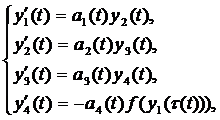where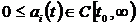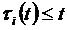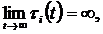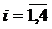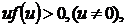(2)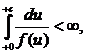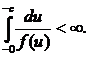The equation

 (3)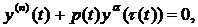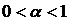is a particular case of this system (sublinear case).

The differential system with the delay of argument in each equation without the condition (2) was studied in this work. The conditions of oscillation of limited solutions for any character of non-linearity (sublinearity, linearity, superlinearity) from results for equation (3) were received.

Let us consider the system

 (4)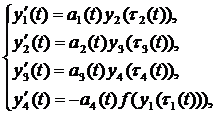where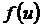– non­decreasing function,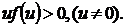The theorem for such system was proven.

Theorem. Let the conditions be executed

1)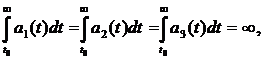(5)

2)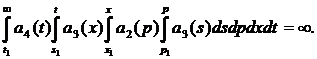Then every solution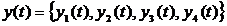of the system (4) oscillates.

The condition (5) takes the form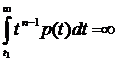for the equation (3).

It`s a sufficient condition for oscillation of limited solutions not only for equations with delay, but also for equations without one.

That`s why the delay has no effect to the oscillating properties of limited solutions.

The list of references:

1. Hryhorzhevskaia A. Y. Study on differential and functional models on finite and infinite intervals / A. Y. Hryhorzhevskaia, N. V. Vareh, M. Y. Atanova // Матеріали Міжнародної студентської науково-практичної конференції «Інно­ва­ційний розвиток держави: проблеми і перспективи очима молодих вчених». – Т. 3: Сучасні дослідження в сфері соціально-гуманітарних наук, Дніпропет­ровськ: ДНУ, 2012. – С. 45–48.